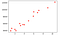# Simple Linear Regression from scratch

The following article explains who you can implement a Simple Linear Regression model from scratch. The motive is to get a good grasp of basic concepts like computing the cost function, implementing gradient descent and using vector notations.

As the name suggests, Simple Linear Regression is one of the most basic Machine Learning algorithms. It is used to model linear relationship between two variables.

Importing necessary packages

• numpy: for representing vectors and statistical computations
• pandas: for reading csv files and
• matplotlib : for plotting and visualization
`import pandas as pdimport numpy as npimport matplotlib.pyplot as plt`

Next, we’ll be loading the dataset. The dataset can be downloaded here. The dataset shows the relationship between the salary of employees and their experience in years.

We skip the first row and read both the columns into separate vectors X and Y.
X contains the years of experience and Y contains the salary.

`X,Y = np.loadtxt("Salary_Data.csv", skiprows=1,unpack=True, delimiter=',')plt.plot(X,Y,'ro')`Scatterplot of the relationship between salary and the number of years of experience

Next we split the dataset into train and test parts. While solving a real problem, we would split the dataset into 3 parts : Training, Validation and Testing sets. But for simplicity, we will train our model using the training set and test its performance on the testing set here.

`from sklearn.model_selection import train_test_splitX_train, X_test, Y_train, Y_test = train_test_split(X, Y, test_size=0.50,random_state=0)plt.plot(X_train,Y_train, 'ro')`

## The hypothesis for Simple Linear Regression is h(x) = θ₀X₀ + θ₁X₁

where x₀=1. Hence we create a new vector `X_one` from `X_train`

`X_one = []for item in X_train:    X_one.append([1, item])`

We have 2 parameters to learn : `theta0` and `theta1`

`theta0 = theta1 = 0theta = np.transpose(np.array([theta0, theta1]))cost = (np.sum((np.dot(X_one, theta) - Y_train)**2))/(2*np.size(X_train))alpha=0.05`

Next, we’ll implement the Gradient Descent Algorithm. It is necessary that you update the values of `theta0` and `theta` simultaneously.

When you update simultaneously, you’re combining the two steps into one step that’s forward and right at the same time. That’s the direction you want to go.

`def gradientDescent(theta0, theta1):# simultaneously updating theta0 and theta1    theta = np.transpose(np.array([theta0, theta1]))    temp0 = theta0 - ((alpha/np.size(X_train)) * (np.sum(np.dot(X_one, theta) - Y_train)) )    temp1 = theta1 - ((alpha/np.size(X_train)) * np.dot((np.dot(X_one, theta) - Y_train), np.transpose(X_train)))    theta0 = temp0    theta1 = temp1    return (theta0, theta1)`

Next we code a cost function to compute the loss for the given hypothesis. Our aim will be to minimize the loss through several iterations.

`def costFunction(theta0, theta1):# returns the cost function for the given value of theta    theta = np.transpose(np.array([theta0, theta1]))    hypothesis = np.dot(X_one, theta)    return (np.sum((hypothesis - Y_train)**2))/(2*np.size(X_train))`

The function `iteration` implements a single iteration of gradient descent and computes the cost using the `costFunction`

`def iteration(theta0, theta1):    (theta0, theta1) = gradientDescent(theta0, theta1)    cost = costFunction(theta0, theta1)    return (cost, theta0, theta1)`

In this implementation, we keep learning till there is no change in the value of theta between the previous and current iterations. You can also let it train for a fixed number of iterations, but implementing it this way would be ideal for the example taken.

`old_theta0 = old_theta1 = 0for i in range (3000):    (cost, theta0, theta1) = iteration(theta0, theta1)    if(theta0 == old_theta0 and theta1 == old_theta1):        break    old_theta0 = theta0; old_theta1 = theta1print(cost, theta0, theta1)`

Now, that we have computed idea values of `theta0` and `theta1` , we use these values to plot a line through the training data.

`plt.plot(X_train,Y_train, 'bo')x = np.linspace(1.1,10.5)y = (theta0) + (theta1)*xplt.plot(x, y, '-r', label='y={} + {}x'.format(theta0, theta1))`

Using the same `theta0` and `theta1` values to plot a line through the testing data. Vamos, the line fits pretty well for the dataset.

`plt.plot(X_test, Y_test, 'bo')x = np.linspace(1.1, 10.5)y = (theta0) + (theta1)*xplt.plot(x, y, '-r' , label='y={} + {}x'.format(theta0, theta1))`

You can get the complete code here

And that’s it. You have implemented a Machine Learning model from scratch.